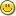### Re: Being stupid - need help

read it and weep(Seems to pass your tests 100% anyway - changing 0-based indexing to 1-based is a bit of a black art)

```-- Calculates the mean squared error
public function MeanSquaredError(sequence truth, prediction)
integer n = length(truth)
end function

public function HoltWinterspredictMult(sequence data, atom alpha, delta, gamma, integer seasonLength)
integer datalen = length(data)
sequence {A,B,S} @= repeat(0.0, datalen)
atom averageFirstSeason = sum(data[1..seasonLength])/seasonLength
B[seasonLength] = averageFirstSeason
S[1..seasonLength] = sq_div(data[1..seasonLength],averageFirstSeason)
for i=seasonLength+1 to datalen do
B[i] = alpha*(data[i]/S[i-seasonLength])+(1-alpha)*(B[i-1]+A[i-1])
A[i] = gamma*(B[i]-B[i-1])+(1-gamma)*A[i-1]
S[i] = delta*(data[i]/B[i])+(1-delta)*S[i-seasonLength]
end for
sequence forecast = repeat(0.0, datalen+seasonLength)
for i=seasonLength+1 to datalen do
forecast[i] = (A[i-1]+B[i-1])*S[i-seasonLength]
end for
for i=datalen+1 to datalen+seasonLength do
forecast[i] = (B[datalen]+(i-datalen)*A[datalen])*S[i-seasonLength]
end for
{A,B,S} @= {}
atom sse = MeanSquaredError(data, forecast)
return {sse, forecast}
end function

public function HoltWinterspredictAdd(sequence data, atom alpha, delta, gamma, integer seasonLength)
integer datalen = length(data)
sequence {A,B,S} @= repeat(0.0, datalen)
atom averageFirstSeason = sum(data[1..seasonLength])/seasonLength
B[seasonLength] = averageFirstSeason
S[1..seasonLength] = sq_sub(data[1..seasonLength],averageFirstSeason)
for i=seasonLength+1 to datalen do
B[i] = alpha*(data[i]-S[i-seasonLength])+(1-alpha)*(B[i-1]+A[i-1])
A[i] = gamma*(B[i]-B[i-1])+(1-gamma)*A[i-1]
S[i] = delta*(data[i]-B[i])+(1-delta)*S[i-seasonLength]
end for
sequence forecast = repeat(0.0, datalen+seasonLength)
for i=seasonLength+1 to datalen do
forecast[i] = A[i-1]+B[i-1]+S[i-seasonLength]
end for
for i=datalen+1 to datalen+seasonLength do
forecast[i] = B[datalen]+((i-datalen)*A[datalen])+S[i-seasonLength]
end for
{A,B,S} @= {}
atom sse = MeanSquaredError(data, forecast)
return {sse, forecast}
end function
```